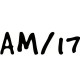Class 10 Maths Notes

# CBSE Class 10 Maths Chapter 10 CirclesWritten by
CirclesCircles

### Circles

A circle is a collection of all points in a plane which are at a constant distance (radius) from a fixed point (centre). We will study Secant & Tangent
Circles are used extensively in our day to day life. Car tyres, football, well, CD, DVDs etc is circular.
Circles are used extensively in our day to day life. Car tyres, football, well, CD, DVDs etc is circular.Circle & a Line
If we consider a circle and a line, there can be three possibilities.
1. A non-intersecting line AB with respect to the circle. In this case there is no common point between line AB & circle
2. Line CD intersecting the circle at one point G. There is only one point G which is common to the line and the circle. In this case, the line CD is called a tangent to the circle.
3. Line EF intersecting the circle at two points H & I. There are two common points H and I that the line EF and the circle have. In this case, we call the line EF a secant of the circle.### Tangent to a Circle

A tangent to a circle is a line that intersects the circle at only one point. The common point G of the tangent and the circle is called the point of contact and the tangent is said to touch the circle at the common point.There is only one tangent at a point of the circle.A circle can have two parallel tangents at the most.
Theorem 1: The tangent at any point of a circle is perpendicular to the radius through the point of contact.
Refer ExamFear video lessons for Proofs.
The line containing the radius through the point of contact is also sometimes called the ‘normal’ to the circle at the point.### Number of tangents from a point on a Circle

If we consider 3 scenarios for the point
1. Point inside circle : 0 tangents
2. Point on the circle : 1 tangent
3. Point outside the circle: 2 tangentsThe length of the segment of the tangent from the external point P and the point of contact with the circle is called the length of the tangent from the point P to the circle. PT1 and PT2 are the lengths of the tangents from P to the circle. The lengths of tangents PT1 and PT2 are equal.
Theorem 2 : The lengths of tangents drawn from an external point to a circle are equal.
Refer ExamFear video lessons for Proofs.
Numerical:  Two concentric circles are of radii 5 cm and 3 cm. Find the length of the chord of the larger circle which touches the smaller circle.
Solution: We have to find length of AC.
∠OBC = 90o, since AC is chord to smaller cirle.
In right triangle OBC, BC2 =OC2 –OB2= 52 -32
Thus BC = 4 cmWe can also prove that Δ OBC ≅ Δ OBA, thus AB = BC
So AB = 2* BC = 2* 4 cm = 8 cm.

### MATHS Revision Notes

Chapter:01  Real Numbers System
Chapter:02  Polynomials
Chapter:03  Pair of Linear Equations in Two Variables
Chapter:05  Arithemetic Progressions
Chapter:06  Triangles
Chapter:07  Coordinate Geometry
Chapter:08  Introduction to Trignometry
Chapter:09  Some Application Of Trignometry
Chapter:10  Circles
Chapter:11  Constructions
Chapter:12  Area Related to Cirles
Chapter:13  Surface Area Volume
Chapter:14  Stastistics
Chapter:15  Probability

Science Revision Notes

English Revision Notes

Economics Revision Notes